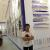QT，在A线程中循环创建N个B线程，经测试，这N个B线程执行完毕之后不会发送finished信号，程序也不会析构它们，何解？

hookover 发布于 2014/07/20 19:55

【福利】CTO直接拍板说好用的SDK全在这里了！0元配齐，全部免费！>>>QT，在A线程中循环创建N个B线程，经测试

public:
A:A(){
//BThread=new B;           //将run（）函数中的new B移动到这里来，B执行完就会正确析构
}
void run(){
while(true){
}
}
private:
}
public:
void run()
{
this->exec();   //写不写都一样
this->quit();     //写不写都一样
}
}

main{
A *a=new A;
a->start();
}

A线程的run函数中不断new出来B线程对像
while(true){
}

-----------------------------------------

————————————————————————————————————————

0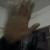while(true)
{//开始
B  b=new B();//这样每次都创建B  结束了就自动析构了他的范围也是在开始和结束的那个大括号里
b->start();
}//结束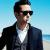0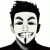It is important to remember that a QThread instance lives in the old thread that instantiated it, not in the new thread that calls run(). This means that all of QThread's queued slots will execute in the old thread. Thus, a developer who wishes to invoke slots in the new thread must use the worker-object approach; new slots should not be implemented directly into a subclassed QThread.
When subclassing QThread, keep in mind that the constructor executes in the old thread while run() executes in the new thread. If a member variable is accessed from both functions, then the variable is accessed from two different threads. Check that it is safe to do so.0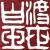this->exec();   //写不写都一样

0A.h

#ifndef A_H
#define A_H

class A : public QThread
{
Q_OBJECT
public:
explicit A(QObject *parent = 0);

signals:

public slots:

protected:
void run();
};

#endif // A_H
A.cpp

#include "a.h"
#include "b.h"
#include <QDebug>

A::A(QObject *parent) :
{
}

void A::run()
{
for (int i = 0 ; i < 10 ; ++i)
{
B* b = new B;
connect(b,&B::finished,[](){
//            if (b->isRunning()) b->quit();
//            b->wait(30); delete b;
qDebug() << "isFinished" << b->isFinished();
qDebug() << "isRunning" <<  b->isRunning();
});
connect(b,&B::finished,b,&B::deleteLater);
b->start();
}
qDebug() << "??";
}
B.h

#ifndef B_H
#define B_H

class B : public QThread
{
Q_OBJECT
public:
explicit B(QObject *parent = 0);
~B();
signals:

public slots:

protected:
void run();
};

#endif // B_H
B.cpp

#include "b.h"
#include <QDebug>

B::B(QObject *parent) :
{
connect(this,&B::finished,[](){qDebug() << "B::finished";});
}

B::~B()
{
qDebug() << "~B : " << QThread::currentThreadId();
}

void B::run()
{
qDebug() << "RUN : " << QThread::currentThreadId();
}
main.cpp

#include <QCoreApplication>
#include "a.h"

int main(int argc, char *argv[])
{
QCoreApplication a(argc, argv);

A aa;
aa.start();

return a.exec();
}~B : 0x9d0 ~B : 0x9d0 ~B : 0x9d0 ~B : 0x9d0 ~B : 0x9d0 ~B : 0x9d0 ~B : 0x9d0 ~B : 0x9d0 ~B : 0x9d0 ~B : 0x9d0 运行结果很神奇0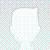A.h的run方法里加入exec(), 在A线程里开启事件循环，用来响应信号槽。

a.h

#ifndef A_H
#define A_H

#include <QVector>
#include "b.h"

class A : public QThread
{
Q_OBJECT
public:
explicit A(QObject *parent = 0);

signals:

public slots:

protected:
void run();

private:
B *b;
};

#endif // A_H
a.cpp

#include "a.h"
#include <QDebug>

A::A(QObject *parent) :
{

}

void A::run()
{
for (int index = 0 ; index < 100 ; ++index)
{
b = new B(NULL, index);
connect(b, SIGNAL(finished()), b, SLOT(deleteLater()));
b->start();
}
qDebug() << "??";
exec();
}

b.h

#ifndef B_H
#define B_H

class B : public QThread
{
Q_OBJECT
public:
explicit B(QObject *parent = 0, int idx = 0);
~B();

signals:

public slots:

protected:
void run();

private:
int index;
};

#endif // B_H
b.cpp

#include "b.h"
#include <QDebug>

B::B(QObject *parent, int idx) :
{
index = idx;
}

B::~B()
{
qDebug() << "~B : " << " index:" << index;
}

void B::run()
{
qDebug() << "RUN : " << " index:" << index;
}
main.cpp

#include <QtCore/QCoreApplication>
#include "a.h"

int main(int argc, char *argv[])
{
QCoreApplication a(argc, argv);
A aa;
aa.start();
return a.exec();
}
xxx.pro

#-------------------------------------------------
#
# Project created by QtCreator 2014-07-21T10:41:14
#
#-------------------------------------------------

QT       += core

QT       -= gui

TARGET = test111
CONFIG   += console
CONFIG   -= app_bundle

TEMPLATE = app

SOURCES += main.cpp \
a.cpp \
b.cpp

a.h \
b.hdanielgone你好， 我想请教一下，A线程使用while循环可以吗？我是希望它一直运行，不停下来，我用信号和槽，来控制变量，使它不断的启动新的线程，释放旧的线程
0A::A(QObject *parent) :
{
connect(this,SIGNAL(signal_run()),this,SLOT(slot_run()));
}

void A::run()
{
int i=0;
while(true)
{
if(i<10)
{
emit signal_run();
i++;
}
}
}

void A::slot_run()
{
B* b = new B;
connect(b,SIGNAL(finished()),b,SLOT(deleteLater()));
b->start();
}0Qt有强大的事件循环呢、、

QRunnable吧0#include<QDebug>

class A : public QRunnable
{
public:
explicit A()
{
}
~A()
{
}
public:
void run()
{
qDebug() << "Run A " << QThread::currentThreadId();
}
};

int main()
{
qDebug() << "expire time out: " << threadPool->expiryTimeout();
for(int i = 0; i < 10; ++i)
{
A *a = new A;
}
{
}
}0a.h

#ifndef A_H
#define A_H

#include <QRunnable>
#include <QObject>
class A : public QObject,  public QRunnable
{
Q_OBJECT
public:
explicit A(QObject *parent = 0);
~A();
void run();

signals:
void runA();

public slots:
void slotRunA();
};

#endif // A_H
a.cpp

#include "a.h"
#include<QDebug>
A::A(QObject *parent) :
QObject(parent)
{
//this->setAutoDelete(true);

}

A::~A()
{
}

void A::run()
{
qDebug() << "RUN A " << QThread::currentThreadId();
emit runA();
}

void A::slotRunA()
{
}
b.h

#ifndef B_H
#define B_H

#include <QObject>
class B : public QObject
{
Q_OBJECT
public:
explicit B(QObject *parent = 0);

signals:

public slots:
void catchRunA();

private:
};

#endif // B_H

b.cpp

#include "b.h"
#include "a.h"

#include <QDebug>
B::B(QObject *parent) :
QObject(parent)
{
qDebug() << "expire time out: " << threadPool->expiryTimeout();
for(int i = 0; i < 10; ++i)
{
A *a = new A;
QObject::connect(a, SIGNAL(runA()), a, SLOT(slotRunA()));
QObject::connect(a, SIGNAL(destroyed()), this, SLOT(catchRunA()));
}

{
}
}

void B::catchRunA()
{
qDebug() << "B catch run a: " << threadPool->activeThreadCount();
}
main.cpp

#include <QCoreApplication>
#include "b.h"
int main(int argc, char** argv)
{
QCoreApplication app(argc, argv);
B b;
return app.exec();
}

0